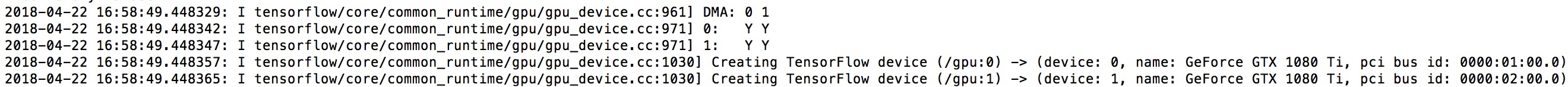# keras 关于使用多个 gpukeras 设置显存 按比例 / 按需 分配

import tensorflow as tf
from keras.backend.tensorflow_backend import set_session
config = tf.ConfigProto()
config.gpu_options.per_process_gpu_memory_fraction = 0.5 #比例
#or
config.gpu_options.allow_growth = True #按需
set_session(tf.Session(config=config))

import os
os.environ["CUDA_VISIBLE_DEVICES"] = "0,1"

CUDA_VISIBLE_DEVICES=0,1 python keras_demo.py

https://www.jianshu.com/p/db0ba022936f

References.

1. 误区
目前Keras是支持了多个GPU同时训练网络，非常容易，但是靠以下这个代码是不行的。

os.environ["CUDA_VISIBLE_DEVICES"] = "1"

1. 目的
为什么要同时用多个GPU来训练？

1. 实现
非常简洁
from model import unet
G = 3 # 同时使用3个GPU
with tf.device("/gpu:0"):
M = unet(input_rows, input_cols, 1)
model = keras.utils.training_utils.multi_gpu_model(M, gpus=G)

model.fit(X_train, y_train,
batch_size=batch_size*G, epochs=nb_epoch, verbose=0, shuffle=True,
validation_data=(X_valid, y_valid))

model.save_weights('/path/to/save/model.h5')
1. 问题
3.1 Compile the model
model.compile(optimizer=optimizer,
loss={'main_output': jaccard_distance_loss, 'aux_output': 'binary_crossentropy'},
metrics={'main_output': jaccard_distance_loss, 'aux_output': 'acc'},
loss_weights={'main_output': 1., 'aux_output': 0.5})

from keras.optimizers import Adam, RMSprop, SGD
model.compile(optimizer=RMSprop(lr=0.045, rho=0.9, epsilon=1.0),
loss={'concatenate_1': jaccard_distance_loss, 'concatenate_2': 'binary_crossentropy'},
metrics={'concatenate_1': jaccard_distance_loss, 'concatenate_2': 'acc'},
loss_weights={'concatenate_1': 1., 'concatenate_2': 0.5})

3.2 Save the model

TypeError: can’t pickle module objects

RuntimeError: Unable to create attribute (object header message is too large)

In https://keras.io/utils/#multi_gpu_model it clearly stated that the model can be used like the normal model, but it cannot be saved, very funny. I can’t even perform reinforced training just because I cannot save the previous model trained with multiple GPUs. If trained with single GPU, the rest of my invested GPUs will become useless. Please urge the developer to look into this bug ASAP.


class CustomModelCheckpoint(keras.callbacks.Callback):

def __init__(self, model, path):
self.model = model
self.path = path
self.best_loss = np.inf

def on_epoch_end(self, epoch, logs=None):
val_loss = logs['val_loss']
if val_loss < self.best_loss:
print("\nValidation loss decreased from {} to {}, saving model".format(self.best_loss, val_loss))
self.model.save_weights(self.path, overwrite=True)
self.best_loss = val_loss

model.fit(X_train, y_train,
batch_size=batch_size*G, epochs=nb_epoch, verbose=0, shuffle=True,
validation_data=(X_valid, y_valid),
callbacks=[CustomModelCheckpoint(model, '/path/to/save/model.h5')])

RuntimeError: Unable to create attribute (Object header message is too large)

model.get_weights(): returns a list of all weight tensors in the model, as Numpy arrays.
model.set_weights(weights): sets the values of the weights of the model, from a list of Numpy arrays. The arrays in the list should have the same shape as those returned by get_weights().

# save model

weight = self.model.get_weights()
np.save(self.path+'.npy', weight)

weight = np.load(load_path)
model.set_weights(weight)

ValueError: You are trying to load a weight file containing 3 layers into a model with 1 layers.

from model import unet
with tf.device("/cpu:0"):
M = unet(input_rows, input_cols, 1)
model = keras.utils.training_utils.multi_gpu_model(M, gpus=G)
model.load_weights(load_path)

#### 当然还有个问题，那就是你使用多模型预测的时候，你可不能注释掉

with tf.device("/cpu:0"):
M = unet(input_rows, input_cols, 1)01-311万+
10-09156
12-07223
09-143万+
07-194442
01-079万+
08-271万+